# RS Aggarwal Solutions for Class 6 Chapter 14 Constructions (Using Ruler and a Pair of Compasses) Exercise 14B

The students can score good marks in a competitive subject like Mathematics by regular practice. The second exercise of chapter 14 has problems which are based on the construction of some special angles using a pair of compasses. A compass is a device which is used for marking the angles in a set of lines. RS Aggarwal Solutions for Class 6 Chapter 14 Constructions (Using Ruler and a Pair of Compasses) Exercise 14B PDF can be downloaded here.

## Download PDF of RS Aggarwal Solutions for Class 6 Chapter 14 Constructions (Using Ruler and a Pair of Compasses) Exercise 14B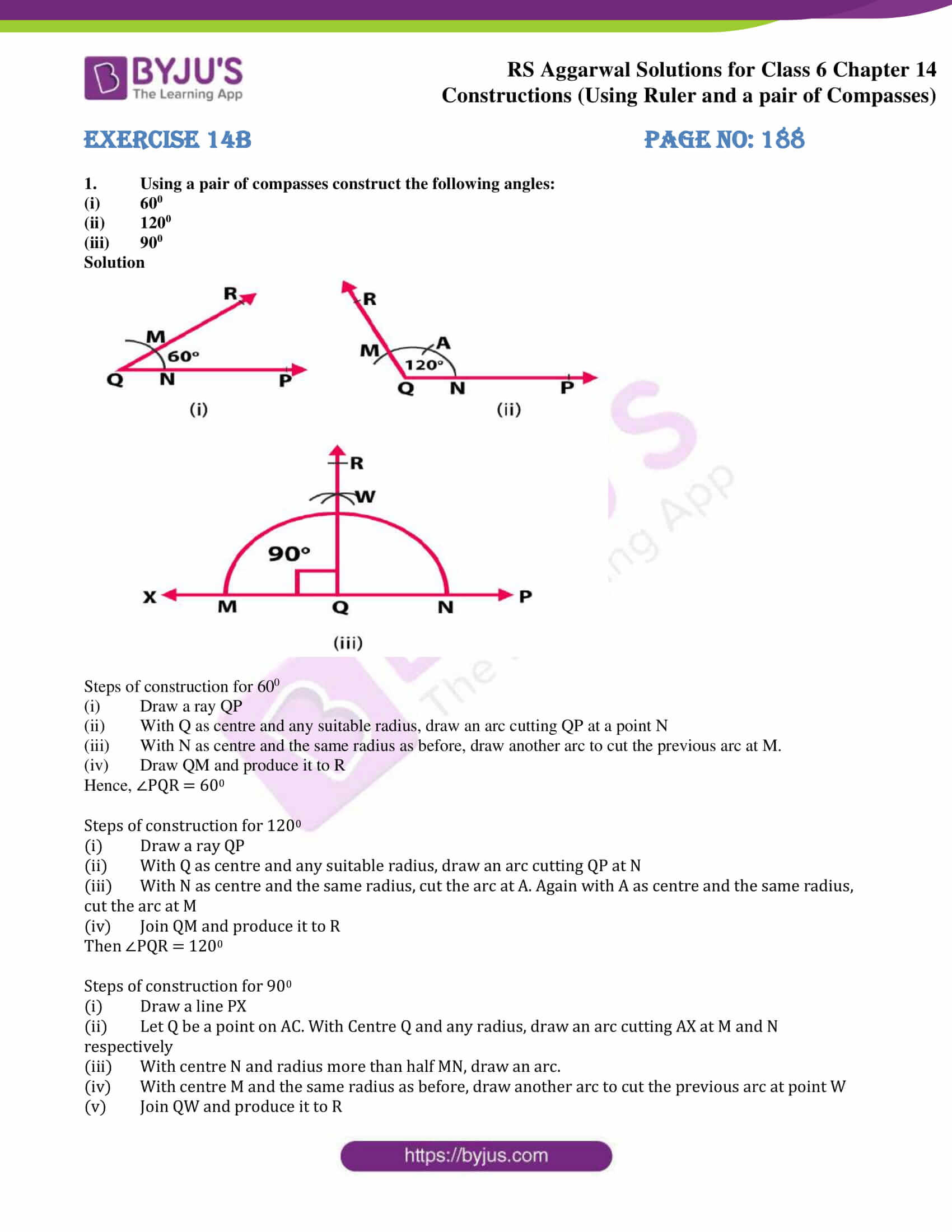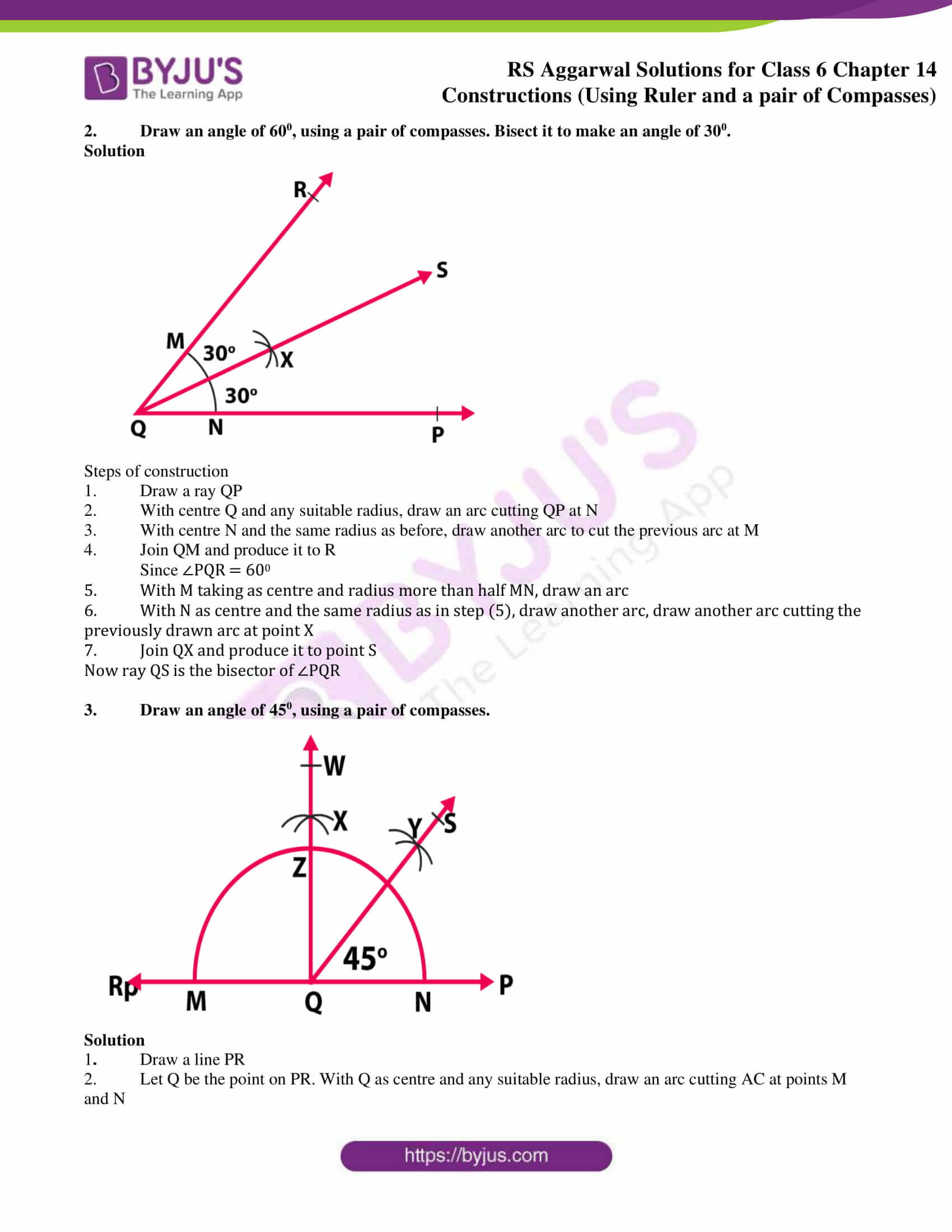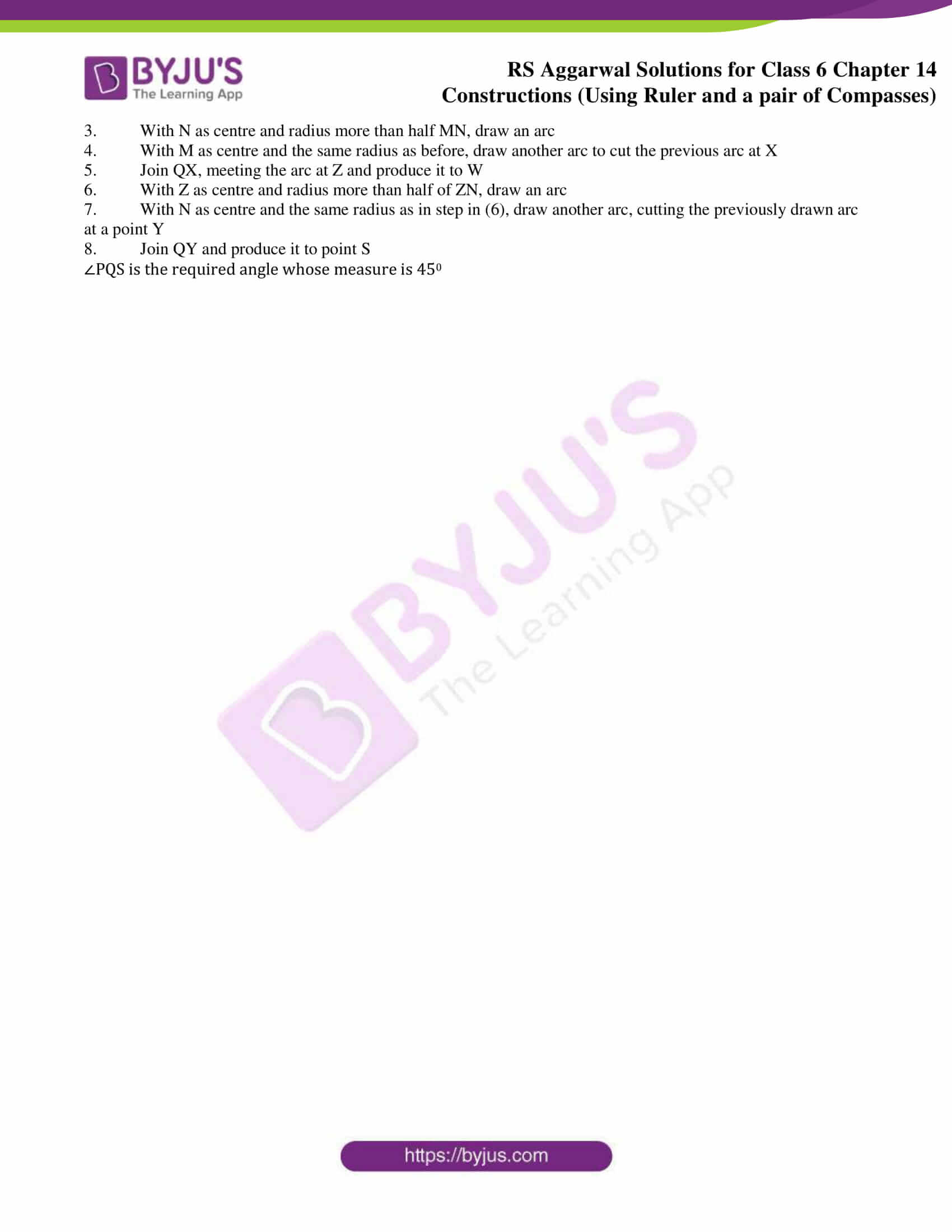### Access answers to Maths RS Aggarwal Solutions for Class 6 Chapter 14 Constructions (Using Ruler and a Pair of Compasses) Exercise 14B

1. Using a pair of compasses construct the following angles:

(i) 600

(ii) 1200

(iii) 900

Solution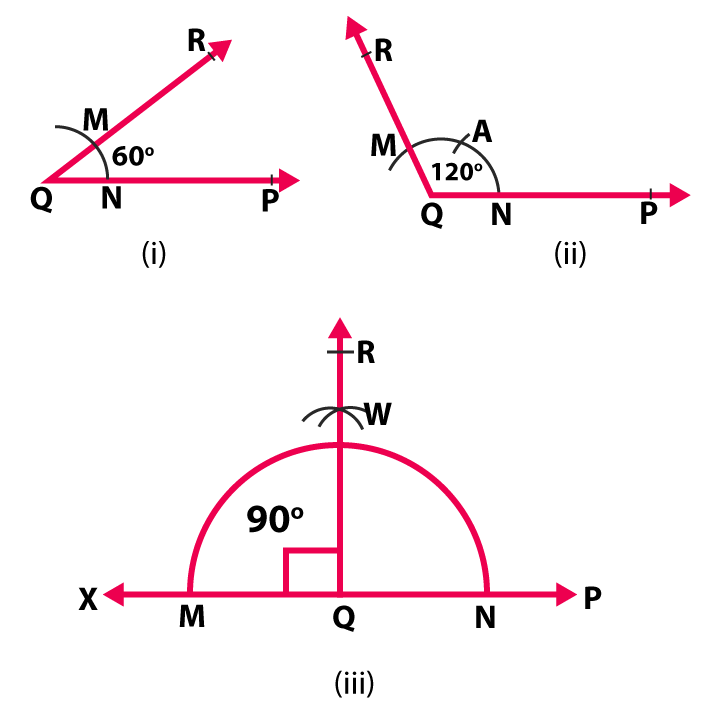Steps of construction for 600

(i) Draw a ray QP

(ii) With Q as centre and any suitable radius, draw an arc cutting QP at a point N

(iii) With N as centre and the same radius as before, draw another arc to cut the previous arc at M.

(iv) Draw QM and produce it to R

Hence, ∠PQR = 600

Steps of construction for 1200

(i) Draw a ray QP

(ii) With Q as centre and any suitable radius, draw an arc cutting QP at N

(iii) With N as centre and the same radius, cut the arc at A. Again with A as centre and the same radius, cut the arc at M

(iv) Join QM and produce it to R

Then ∠PQR = 1200

Steps of construction for 900

(i) Draw a line PX

(ii) Let Q be a point on AC. With Centre Q and any radius, draw an arc cutting AX at M and N respectively

(iii) With centre N and radius more than half MN, draw an arc.

(iv) With centre M and the same radius as before, draw another arc to cut the previous arc at point W

(v) Join QW and produce it to R

2. Draw an angle of 600, using a pair of compasses. Bisect it to make an angle of 300.

Solution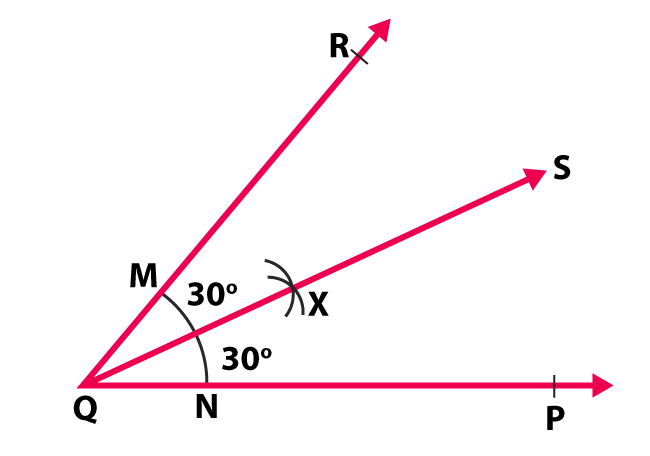Steps of construction

1. Draw a ray QP

2. With centre Q and any suitable radius, draw an arc cutting QP at N

3. With centre N and the same radius as before, draw another arc to cut the previous arc at M

4. Join QM and produce it to R

Since ∠PQR = 600

5. With M taking as centre and radius more than half MN, draw an arc

6. With N as centre and the same radius as in step (5), draw another arc, draw another arc cutting the previously drawn arc at point X

7. Join QX and produce it to point S

Now ray QS is the bisector of ∠PQR

3. Draw an angle of 450, using a pair of compasses.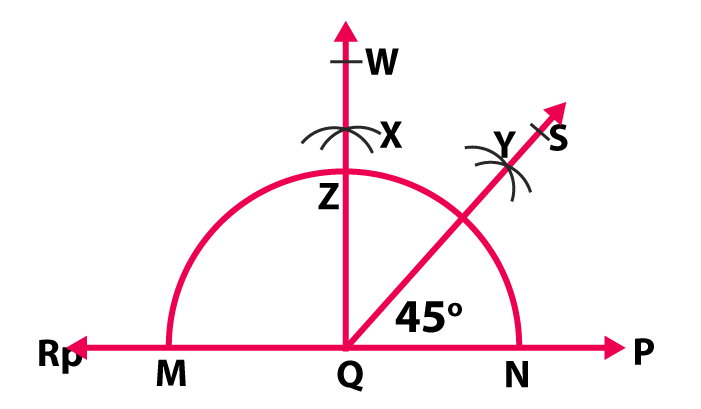Solution

1. Draw a line PR

2. Let Q be the point on PR. With Q as centre and any suitable radius, draw an arc cutting AC at points M and N

3. With N as centre and radius more than half MN, draw an arc

4. With M as centre and the same radius as before, draw another arc to cut the previous arc at X

5. Join QX, meeting the arc at Z and produce it to W

6. With Z as centre and radius more than half of ZN, draw an arc

7. With N as centre and the same radius as in step in (6), draw another arc, cutting the previously drawn arc at a point Y

8. Join QY and produce it to point S

∠PQS is the required angle whose measure is 450

### Access other exercises of RS Aggarwal Solutions for Class 6 Chapter 14 Constructions (Using Ruler and a Pair of Compasses)

Exercise 14A Solutions